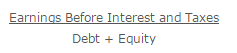Accounting

# Return on Total Capital

The Return on Total Capital compares the profitability of a business to the aggregate amount of funds invested in it. The concept is most applicable to businesses that use large amounts of debt in their capital structure. These entities employ leverage to achieve a high return on equity. To see how they are performing when using all forms of funding, we use the return on total capital.

The formula for the return on total capital is to divide earnings before interest and taxes by the aggregate amount of debt and equity. The calculation is:For example, a business has generated \$150,000 of earnings before interest and taxes. As of the end of the reporting period, it has \$300,000 of debt and \$700,000 of equity. Its return on total capital is:The measurement can be altered to use operating profit, if there are stray profitability results from financing and other activities that are materially skewing the results. For example, there may be a large amount of derivative-based income that masks an operating loss.

Example

Return on invested capital (ROIC) is a profitability ratio. It measures the return that an investment generates for those who have provided capital, i.e. bondholders and stockholders. ROIC tells us how good a company is at turning capital into profits.

How it works

The general equation for ROIC is: ( Net income – Dividends ) / ( Debt + Equity )

ROIC can also be known as “return on capital” or “return on total capital.”

For example, Manufacturing Company MM lists \$100,000 as net income, \$500,000 in total debt and \$100,000 in shareholder equity. Its business operations are straightforward — MM makes and sells widgets.

We can calculate MM’s ROIC with the equation:

ROIC = ( Net income – Dividends ) / ( Debt + Equity )

= (100,000 – 0) / (500,000 + 100,000) = 16.7%

Note that for some companies, net income may not be the profitability measure you want to use. You want to make sure that the profit metric you put in the numerator is giving you the information you need.